Join one of our upcoming courses taking place around the world! Explore now

# Pile Types

The Define Pile Types option is available through Piles > Pile Types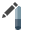.Pile Types are made up of Pile Sections whose properties are defined in the Pile Section Properties dialog.

The properties and options available for a pile type are dependent on the analysis option selected, as is explained in the table below.

 RSPile Pile Section Properties Pile Type Analysis Cross-Section Material Type Material Properties Multiple Sections Bell Section* Tapered Section Driven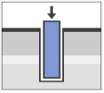Pipe Pipe Closed End Not Applicable Not Required YES NO YES Pile Pipe Open End YES NO YES Timber YES NO YES Concrete Pile YES NO YES H Pile YES NO NO BoredCircular Concrete Concrete strength YES YES NO Square YES NO NO Rectangular YES NO NO Axial/Lateral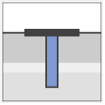Circular Elastic Modulus YES YES YES Rectangular YES NO YES Pipe YES NO YES Typical Section YES NO NO User-Defined YES NO NO Circular Plastic Modulus Mxy Mxz YES YES YES Rectangular YES NO YES Pipe YES NO YES Circular Reinforced Concrete Concrete strength YES YES NO Rectangular YES NO NO Circular Prestressed Concrete Concrete strength YES NO NO Rectangular YES NO NO

#### Notes:

• Group analysis has the same Pile Type features as Lateral/Axial analysis
• *Bell Section cannot exist by itself and can only be added as the bottom-most section
• Skin friction is ignored along a Bell Section
• P-Y and Q-Z curves of a bell section are obtained using the actual bell dimensions
• The structural stiffness of a bell section is calculated using the stem diameter
• The bell obtains its properties from the section immediately above it including any reinforcement design

The Edit Pile Types dialog is separated into two sections which are explained below:

• Sections
• Orientation

## Pile Sections

The Section tab allows users to construct a Pile Type using Pile Sections. In this tab users can define the:

• Cross Section Type
• Number of Sections
• Length of each Section
• Bell geometry and tapering

### BELLS

Depending on the type of analysis being done, a bell can be added and defined using the Design Bell option.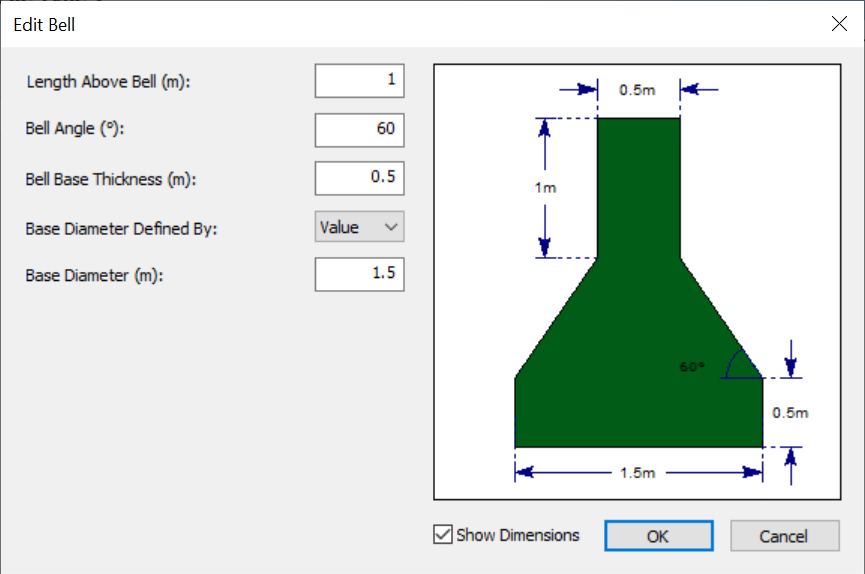In this dialog users can define:

• Length Above Bell
• Bell Angle
• Bell Base Thickness
• Base Diameter

When adding a bell it should be noted:

• Bell Sections cannot exist by themselves and can only be added as the bottom-most section of the pile type
• Skin friction is ignored along a Bell Section
• P-Y and Q-Z curves of a bell section are obtained using the actual bell dimensions
• The structural stiffness of a bell section is calculated using the stem diameter
• The bell obtains its properties from the section immediately above it including any reinforcement design

#### Assumptions for different analysis options

Certain assumptions are made for each type of analysis when a bell is added to a pile type:

Lateral

• Skin friction is ignored along a bell section
• P-Y curves along a bell section are obtained using the interpolated bell diameter

Axial

• T-Z is ignored along a bell section
• Q-Z is obtained using the bell base diameter
• The structural stiffness of a bell section is computed using the stem diameter

Bored Analysis

• Skin friction is ignored along a bell section
• Pile capacity along a bell section is obtained using the interpolated bell diameter

## Orientation

The orientation inputs are used to specify the pile batter and orientation in 3D space. Two options are available:

• Alpha/Beta
• Alpha - Rotation of pile around z-axis, clockwise from the y-axis
• Beta - Batter angle of pile, clockwise from y-axis
• Theta - Rotation of pile around the central axis of the pile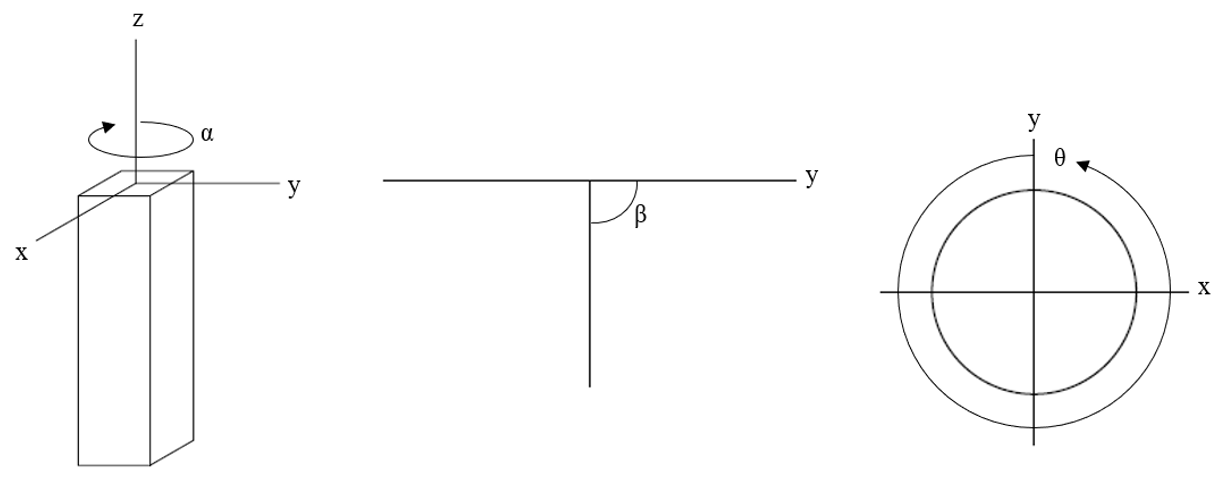From left: alpha, beta, theta

• Vector
• XYZ - Direction vector coordinates. The normalized unit vector of the input vector is calculated.
• Rotation Angle - the angle of rotation (clockwise, in degrees) of the pile section about the z-axis. (0 degrees if no rotation).

#### Notes on Ground Slope and Pile Batter

• Ground Slope and Pile Batter are only one of the options for defining a battered pile / sloping ground. T-z and Q-z multipliers can be used to analyze axially loaded (battered) piles in sloping ground. Similarly, p-y multipliers are more commonly used to analyze laterally loaded (battered) piles in sloping ground.